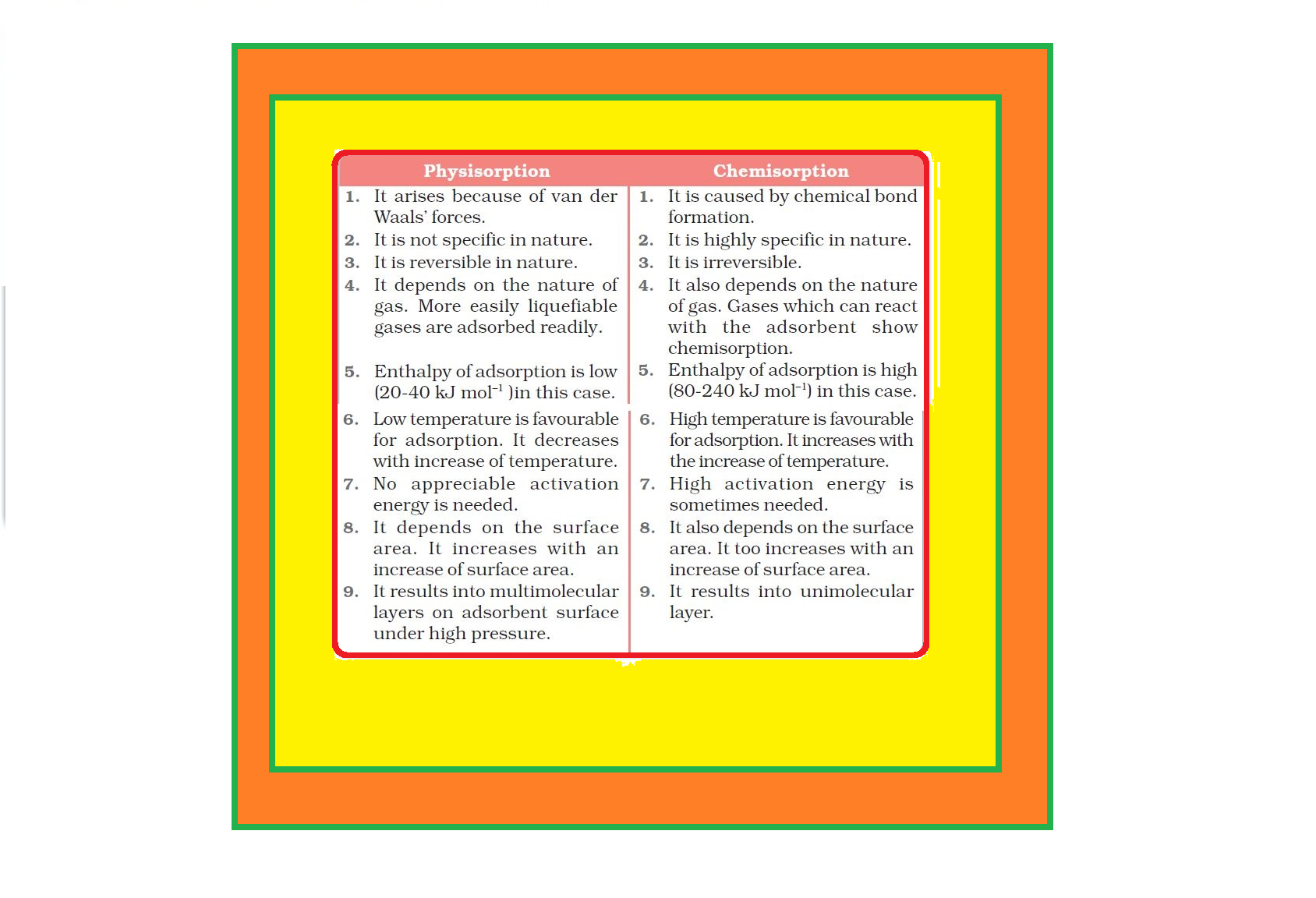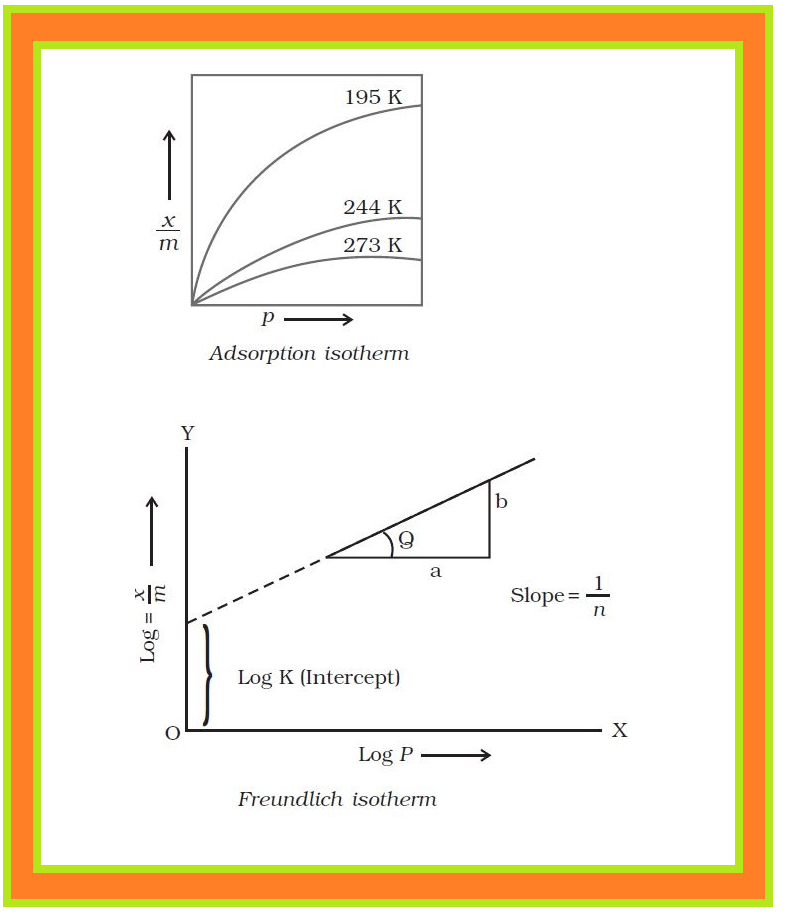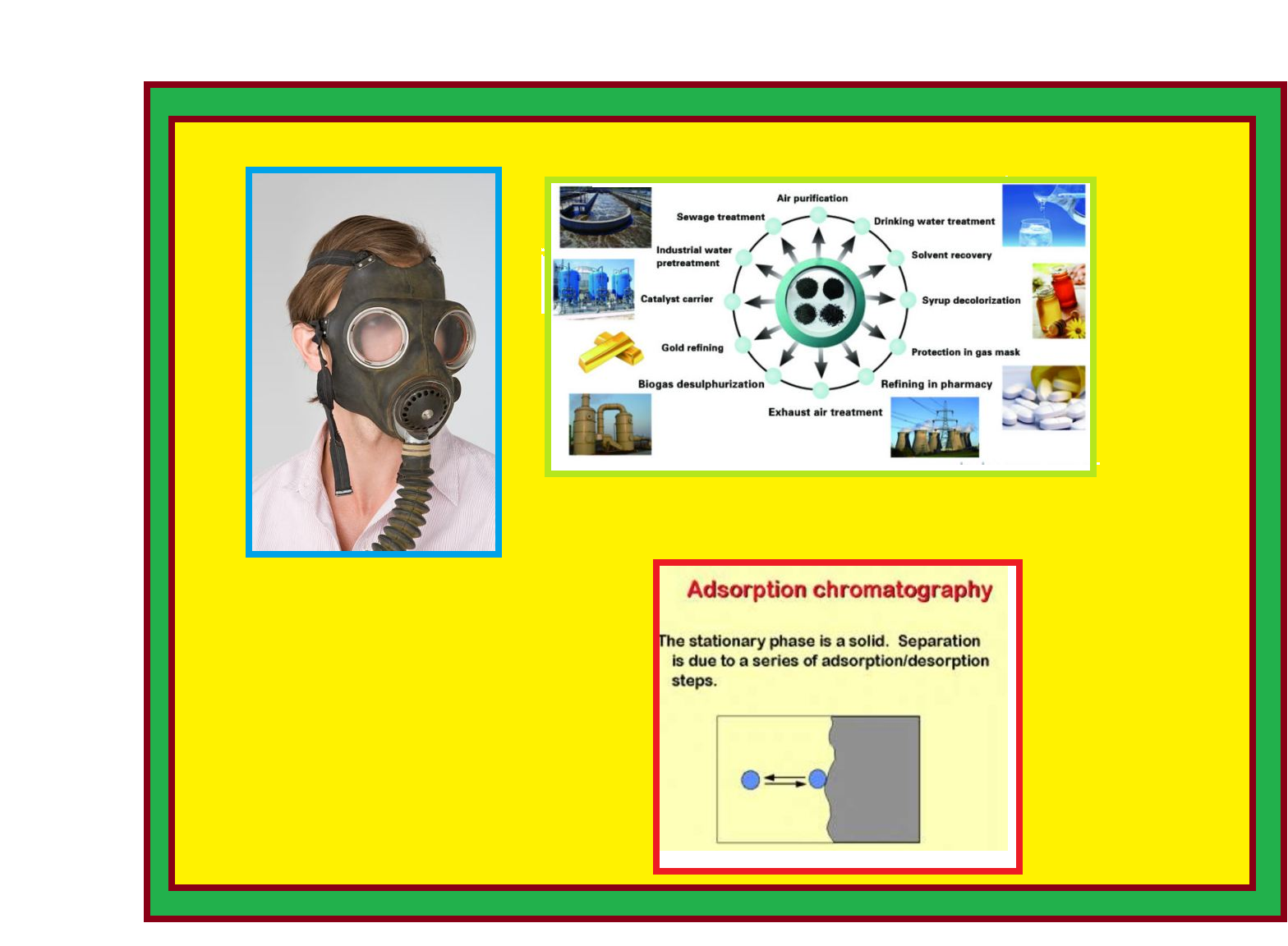Chemistry Types of Adsorption, Adsorption Isotherm and Application
Click for Only Video

### Topics Covered :

● Types of Adsorption
● Characteristics of Physisorption
● Characteristics of Chemisorption
● Adsorption Isotherm
● Adsorption from Solution Phase
● Applications of Adsorption

### Types of Adsorption :

=> There are mainly two types of adsorption of gases on solids.

(i) text(Physisorption) : If accumulation of gas on the surface of a solid occurs on account of weak van der Waals’ forces, the adsorption is termed as physical adsorption or physisorption.

(ii) text(Chemisorption) : When the gas molecules or atoms are held to the solid surface by chemical bonds, the adsorption is termed chemical adsorption or chemisorption.

● The chemical bonds may be covalent or ionic in nature.

● Chemisorption involves a high energy of activation and is, therefore, often referred to as activated adsorption.

=> Sometimes these two processes occur simultaneously and it is not easy to ascertain the type of adsorption.

=> A physical adsorption at low temperature may pass into chemisorption as the temperature is increased.

● For example, dihydrogen is first adsorbed on nickel by van der Waals’ forces. Molecules of hydrogen then dissociate to form hydrogen atoms which are held on the surface by chemisorption.

### Characteristics of Physisorption :

(i) text(Lack of specificity :) A given surface of an adsorbent does not show any preference for a particular gas as the van der Waals’ forces are universal.

(ii) text(Nature of Adsorbate :) The amount of gas adsorbed by a solid depends on the nature of gas.

● In general, easily liquefiable gases (i.e., with higher critical temperatures) are readily adsorbed as van der Waals’ forces are stronger near the critical temperatures.

● Therefore, 1g of activated charcoal adsorbs more sulphur dioxide (critical temperature 630K), than methane (critical temperature 190K) which is still more than 4.5 mL of dihydrogen (critical temperature 33K).

(iii) text(Reversible Nature :) Physical adsorption of a gas by a solid is generally reversible. Thus,

Solid + Gas l Gas/Solid + Heat

● More of gas is adsorbed when pressure is increased as the volume of the gas decreases (Le-Chateliers’s principle) and the gas can be removed by decreasing pressure.

● Since the adsorption process is exothermic, the physical adsorption occurs readily at low temperature and decreases with increasing temperature (Le-Chatelier’s principle).

(iv) text(Surface Area of Adsorbent :) The extent of adsorption increases with the increase of surface area of the adsorbent. Thus, finely divided metals and porous substances having large surface areas are good adsorbents.

(v) text(Enthalpy of Adsorption :) Physical adsorption is an exothermic process but its enthalpy of adsorption is quite low (20– 40 kJ mol^(-1)). This is because the attraction between gas molecules and solid surface is only due to weak van der Waals’ forces.

### Characteristics of Chemisorption :(i) text(High Specificity :) Chemisorption is highly specific and it will only occur if there is some possibility of chemical bonding between adsorbent and adsorbate.

● For example, oxygen is adsorbed on metals by virtue of oxide formation and hydrogen is adsorbed by transition metals due to hydride formation.

(ii) text(Irreversibility :) As chemisorption involves compound formation, it is usually irreversible in nature. Chemisorption is also an exothermic process but the process is very slow at low temperatures on account of high energy of activation.

● Like most chemical changes, adsorption often increases with rise of temperature.

● Physisorption of a gas adsorbed at low temperature may change into chemisorption at a high temperature. Usually high pressure is also favourable for chemisorption.

(iii) text(Surface Area :) Like physical adsorption, chemisorption also increases with increase of surface area of the adsorbent.

(iv) text(Enthalpy of Adsorption :) Enthalpy of chemisorption is high (80-240 kJ mol^(-1)) as it involves chemical bond formation.

### Adsorption Isotherms :text(Definition) : The variation in the amount of gas adsorbed by the adsorbent with pressure at constant temperature can be expressed by means of a curve termed as adsorption isotherm.

text(Freundlich Adsorption Isotherm :) Freundlich, in 1909, gave an empirical relationship between the quantity of gas adsorbed by unit mass of solid adsorbent and pressure at a particular temperature. The relationship can be expressed by the following equation :

x/m = kp^(1/n) ( n > 1) ...........(1).

● where x is the mass of the gas adsorbed on mass m of the adsorbent at pressure P,

● k and n are constants which depend on the nature of the adsorbent and the gas at a particular temperature.

=> The relationship is generally represented in the form of a curve where mass of the gas adsorbed per gram of the adsorbent is plotted against pressure.

=> These curves indicate that at a fixed pressure, there is a decrease in physical adsorption with increase in temperature. These curves always seem to approach saturation at high pressure.

Taking logarithm of eq. (1)

log \ \ x/m = log k +1/n log P .................(2)

=> The validity of Freundlich isotherm can be verified by plotting log \ \ x/m on y-axis (ordinate) and log P on x-axis (abscissa).

● If it comes to be a straight line, the Freundlich isotherm is valid, otherwise not.

● The slope of the straight line gives the value of 1/n. The intercept on the y-axis gives the value of log k.

=> Freundlich isotherm explains the behaviour of adsorption in an approximate manner.

=> The factor 1/n can have values between 0 and 1 (probable range 0.1 to 0.5). Thus, equation (2) holds good over a limited range of pressure.

● when 1/n = 0 , x/m =  constant, the adsorption is independent of pressure.

● when 1/n = 1 , x/m = k P ,  i.e x/m prop P the adsorption varies directly with pressure.

Both the conditions are supported by experimental results. The experimental isotherms always seem to approach saturation at high pressure. This cannot be explained by Freundlich isotherm. Thus, it fails at high pressure.

### Adsorption from Solution Phase :

=> Solids can adsorb solutes from solutions also.

● When a solution of acetic acid in water is shaken with charcoal, a part of the acid is adsorbed by the charcoal and the concentration of the acid decreases in the solution.

● In the same way, the litmus solution when shaken with charcoal becomes colourless.

● The precipitate of Mg(OH)_2 attains blue colour when precipitated in presence of magneson reagent. The colour is due to adsorption of magneson.

=> The following observations have been made in the case of adsorption from solution phase :

(i) The extent of adsorption decreases with an increase in temperature.

(ii) The extent of adsorption increases with an increase of surface area of the adsorbent.

(iii) The extent of adsorption depends on the concentration of the solute in solution.

(iv) The extent of adsorption depends on the nature of the adsorbent and the adsorbate.

=> The precise mechanism of adsorption from solution is not known.

=> Freundlich’s equation approximately describes the behaviour of adsorption from solution with a difference that instead of pressure, concentration of the solution is taken into account, i.e.,

x/m = k C^(1/n) ............(3)

(C is the equilibrium concentration, i.e., when adsorption is complete). On taking logarithm of the above equation, we have

log \ \ x/m = log k +1/n logC .........(4)

=> Plotting log \ \ x/m against log C a straight line is obtained which shows the validity of Freundlich isotherm.

=> This can be tested experimentally by taking solutions of different concentrations of acetic acid. Equal volumes of solutions are added to equal amounts of charcoal in different flasks. The final concentration is determined in each flask after adsorption. The difference in the initial and final concentrations give the value of x. Using the above equation, validity of Freundlich isotherm can be established.

### Applications of Adsorption :The phenomenon of adsorption finds a number of applications. Important ones are listed here :

(i) text(Production of High Vacuum :) The remaining traces of air can be adsorbed by charcoal from a vessel evacuated by a vacuum pump to give a very high vacuum.

(ii) text(Gas Masks :) Gas mask (a device which consists of activated charcoal or mixture of adsorbents) is usually used for breathing in coal mines to adsorb poisonous gases.

(iii) text(Control of Humidity :) Silica and aluminium gels are used as adsorbents for removing moisture and controlling humidity.

(iv) text(Removal of Colouring Matter from Solutions :) Animal charcoal removes colours of solutions by adsorbing coloured impurities.

(v) text(Heterogeneous Catalysis :) Adsorption of reactants on the solid surface of the catalysts increases the rate of reaction. There are many gaseous reactions of industrial importance involving solid catalysts. Manufacture of ammonia using iron as a catalyst,
manufacture of H_2SO_4 by contact process and use of finely divided nickel in the hydrogenation of oils are excellent examples of heterogeneous catalysis.

(vi) text(Separation of Inert Gases :) Due to the difference in degree of adsorption of gases by charcoal, a mixture of noble gases can be separated by adsorption on coconut charcoal at different temperatures.

(vii) text(In Curing Diseases :) A number of drugs are used to kill germs by getting adsorbed on them.

(viii) text(Froth Floatation Process :) A low grade sulphide ore is concentrated by separating it from silica and other earthy matter by this method using pine oil and frothing agent.

(ix) text(Adsorption Indicators :) Surfaces of certain precipitates such as silver halides have the property of adsorbing some dyes like eosin, fluorescein, etc. and thereby producing a characteristic colour at the end point.

(x) text(Chromatographic Analysis :) Chromatographic analysis based on the phenomenon of adsorption finds a number of applications in analytical and industrial fields.